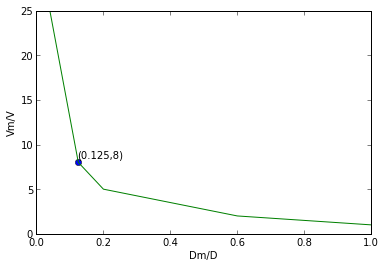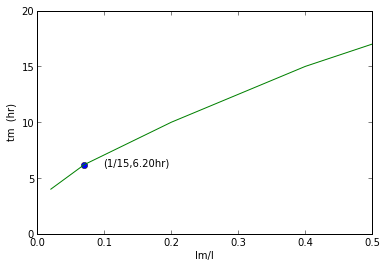# Chapter 7 :Similitude ,Dimensional Analysis and Modelling¶

### Example 7.5 Page no.372¶

In :
D=0.1                            #m
H=0.3                            #m
v=50.0                           #km/hr
Dm=20.0                          #mm
T=20.0                           #degree C
fm=49.9                          #Hz  frequency for the model
#f=func(D,H,V,d,vis)
#f=T**(-1) D=l H=L V=L*(T**(-1)) d=M*(L**(-3)) vis=M*(L**(-1))*(T**(-1))
#by applying pi theorem,
#(f*D/V)=funct((D/H),(d*V*D/vis))
#hence Dm/Hm = D/H, dm*Vm*Dm/vism = d*V*D/vis, and (f*D/V)=(fm*Dm/Vm)

#calculation
Hm=(Dm*H*1000/(D*1000))   #mm
V=v*1000/3600             #m/s
vism=0.001                #kg/(m*s)
vis=1.79*10**-5           #kg/(m*s)
d=1.23                        #kg/(m**3)
dm=998                        #kg/(m**3)
Vm=(vism*d*D*V*1000)/(vis*dm*Dm)        #m/s
f=(V/Vm)*(Dm/(D*1000))*fm               #Hz

#result
print "The model dimension =",round(Hm,3),"mm"
print "The velocity at which the test should be performed=",round(Vm,2),"m/s"
print "The predicted prototype vortex shredding frequency =",round(f,1),"Hz"

The model dimension = 60.0 mm
The velocity at which the test should be performed= 4.781 m/s
The predicted prototype vortex shredding frequency = 29.0 Hz


### Example 7.6 Page no.377¶

In :
%matplotlib inline

Welcome to pylab, a matplotlib-based Python environment [backend: module://IPython.zmq.pylab.backend_inline].

In :
D=2.0                              #ft
Q=30.0                           #cfs
Dm=3.0                          #in
#Rem=Re hence (Vm*Dm/kvism)=(V*D/kvis) where kvis is kinematic viscosity
#kvis=kvism same fluid is used for model and prototype
#(Vm/V)=(D/Dm)
#Q=VA hence Qm/Q = (Vm*Am)/(V*A)=(Dm/D)

#  calculation
Qm=(Dm/12)*Q/D          #cfs

#result
print "The required flowrate in the model=",Qm,"cfs"

#Plot
import matplotlib.pyplot as plt
fig = plt.figure()
ax.plot([0.125], , 'o')
ax.annotate('(0.125,8)', xy=(0.125,8.5))

T=[0.04,0.125,0.2,0.6,1]
K=[25,8,5,2,1]
xlabel("Dm/D")
ylabel("Vm/V")
plt.xlim((0,1))
plt.ylim((0,25))
a=plot(T,K)
show(a)

The required flowrate in the model= 3.75 cfs### Example 7.7 Page no.380¶

In :
V=240.0                             #mph
ratio=0.1
Vair=240.0                          #mph
Fm=1.0                               #lb Fm =drag force on model
p=14.7                              #psia standard atmospheric pressure
#Re=Rem
#(d*V*l/vis)=(dm*Vm*lm/vism)
#here Vm=V and lm/l=ratio
#assumption made is that an increase in pressure does not significantly change viscosity

#calculation
drat=V/(ratio*Vair)             #where drat=dm/d

#for an ideal gas p=d*R*T
#T=Tm
#hence, pm/p=dm/d pm/p=prat
pm=p*drat
#F/(0.5*d*(V**2)*(l**2))=Fm/(0.5*dm*(Vm**2)*(lm**2))
F=(1/drat)*((V/Vair)**2)*((1/ratio)**2)*Fm

#result
print "The required air pressure in the tunnel=",round(pm,3),"psia"
print "The corrosponding drag on the prtotype \nfor a 1 lb drag on the model=",round(F,3),"lb"

The required air pressure in the tunnel= 147.0 psia
The corrosponding drag on the prtotype
for a 1 lb drag on the model= 10.0 lb


### Example 7.8 Page no.384¶

In :
w=20.0                           #m
Q=125.0                        #(m**3)/s
ratio=0.066
t=24.0                           #hours
wm=ratio*w              #m
#Vm/(gm*lm)**0.5 = V/(g*l)**0.5
#gm=g
#Q=VA and lm/l=1/15
#hence Qm/Q = ((lm/l)**0.5)*((lm/l)**2) = ratio**2.5
Qm=(ratio**2.5)*Q
#V=l/t
#tm/t=(V/Vm)*(lm/l)=ratio**0.5
tm=(ratio**0.5)*t         #hours

#result
print "The required model width=",round(wm,3),"m"
print "The required model flowrate=",round(Qm,3),"m**3/s"
print "The operating time for the model=",round(tm,1),"hrs"

#plot
import matplotlib.pyplot as plt
fig = plt.figure()

T=[0.02,0.07,0.2,0.4,0.5]
K=[4,6.20,10,15,17]
xlabel("lm/l")
ylabel("tm  (hr)")
plt.xlim((0,0.5))
plt.ylim((0,20))

ax.plot([0.07], [6.20], 'o')
ax.annotate('(1/15,6.20hr)', xy=(0.1,6))
a=plot(T,K)
show(a)

The required model width= 1.32 m
The required model flowrate= 0.14 m**3/s
The operating time for the model= 6.2 hrs